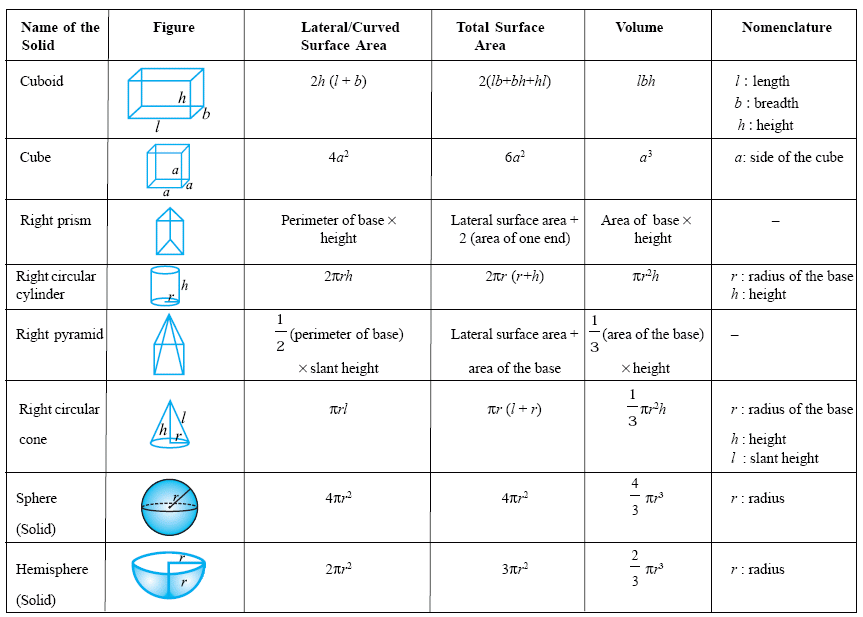Email us to get an instant 20% discount on highly effective K-12 Math & English kwizNET Programs!

Online Quiz (WorksheetABCD)

Questions Per Quiz = 2 4 6 8 10

High School Mathematics4.1 Geometry Formulas SummaryAreas

square = a2
rectangle = ab
circle = p r2
ellipse = p r1 r2
triangle = (1/2) b h
equilateral triangle = Ö3/4 a2
triangle given SAS = (1/2) a b sin C
triangle given a,b,c = Ö[s(s-a)(s-b)(s-c)]
when s = (a+b+c)/2 (Heron's formula)
parallelogram = bh
trapezoid = h/2 (b1 + b2)
regular polygon = (1/2) n sin(360°/n) a2
when n = # of sides and a = length from center to a corner

Volumes

cube = a3
rectangular prism = a b c
irregular prism = b h
cylinder = b h = p r2h
pyramid = (1/3) b h
cone = (1/3) b h = 1/3 [p r2 h ]
sphere = (4/3) p r3
ellipsoid = (4/3) p r1 r2 r3
Directions: Memorize the above formulae and have someone test your knowledge. Write all the formulas on a sheet of paper.